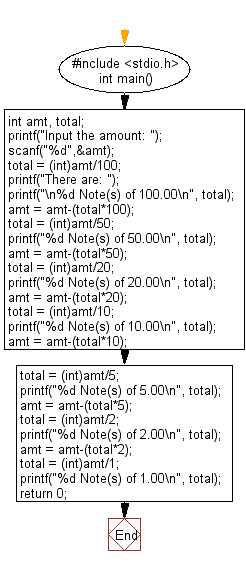﻿ C : Break an amount into the smallest number of banknotes

# C Exercises: Read an amount and break the amount into smallest possible number of bank notes

## C Basic Declarations and Expressions: Exercise-16 with Solution

Write a C program to read an amount (integer value) and break the amount into the smallest possible number of bank notes.
Note: The possible banknotes are 100, 50, 20, 10, 5, 2 and 1.

C Code:

``````#include <stdio.h>
int main() {
int amt, total; // Declare variables for amount and total

// Prompt user for the amount and store it in 'amt'
printf("Input the amount: ");
scanf("%d",&amt);

// Calculate and print the number of each denomination
total = (int)amt/100;
printf("There are:\n");
printf("%d Note(s) of 100.00\n", total);
amt = amt-(total*100);

total = (int)amt/50;
printf("%d Note(s) of 50.00\n", total);
amt = amt-(total*50);

total = (int)amt/20;
printf("%d Note(s) of 20.00\n", total);
amt = amt-(total*20);

total = (int)amt/10;
printf("%d Note(s) of 10.00\n", total);
amt = amt-(total*10);

total = (int)amt/5;
printf("%d Note(s) of 5.00\n", total);
amt = amt-(total*5);

total = (int)amt/2;
printf("%d Note(s) of 2.00\n", total);
amt = amt-(total*2);

total = (int)amt/1;
printf("%d Note(s) of 1.00\n", total);

return 0;
}
``````

Explanation:

In the exercise above -

• First, the program includes the standard library for input/output functions.
• It declares the following variables:
• int amt: This variable stores the input amount.
• int total: This variable will be used to calculate the number of each denomination of currency notes.
• The program prompts the user to input the amount:
• It uses "printf()" function to display "Input the amount: " to instruct the user to input the amount.
• It uses "scanf()" function to read the user's input and store it in the variable amt.
• The program calculates the number of each denomination of currency notes:
• Starting with the largest denomination and moving to the smallest, it calculates the number of notes needed for each denomination.
• For each denomination (e.g., 100, 50, 20, etc.), it calculates the number of notes and updates the 'total' variable accordingly.
• After calculating the number of notes for each denomination, it subtracts the total value of those notes from the remaining amount (amt) to continue with the next denomination.
• The program uses "printf()" function to display the number of notes for each denomination:
• It prints the number of notes for each denomination, using the 'total' variable.
• The format is "X Note(s) of Y.YY" where X is the number of notes, and Y.YY is the denomination.
• Finally, the program returns 0 to indicate successful execution.

Sample Output:

```Input the amount: 375
There are:
3 Note(s) of 100.00
1 Note(s) of 50.00
1 Note(s) of 20.00
0 Note(s) of 10.00
1 Note(s) of 5.00
0 Note(s) of 2.00
0 Note(s) of 1.00
```

Flowchart:C Programming Code Editor:

What is the difficulty level of this exercise?

Test your Programming skills with w3resource's quiz.

﻿